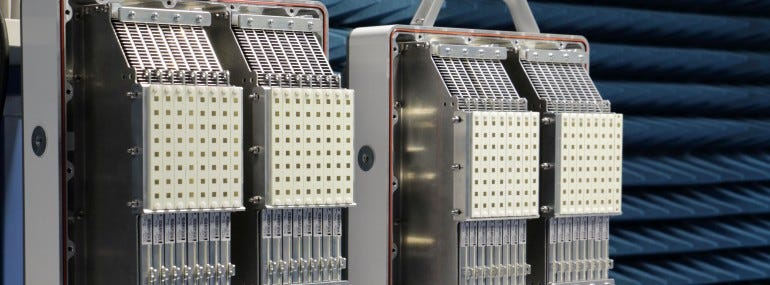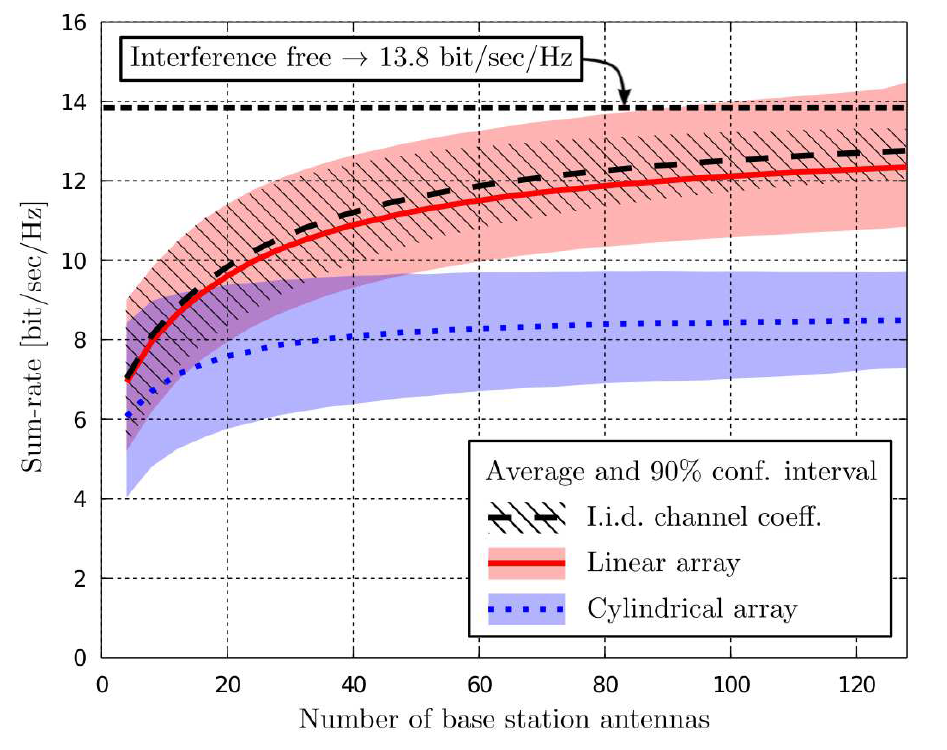# Massive MIMO

## MIMO with large phased array antenna array at the base station64 TX + 64 RX 5G MU-MIMO antenna suitable for Massive MIMO (Credit: Ericsson)

Multiple Input Multiple Output (MIMO) techniques are used in wireless system to direct the antenna beam towards the user. This improves the antenna gain and reduces interference towards other users.

Typically, MIMO is implemented with multiple antennas at the base station and the mobile. 5G systems take the MIMO concept even further by adding a very large number of antennas at the base station. This is referred to as massive MIMO.

# Massive MIMO theory

## System model

Consider the system show below, two mobiles UE1 and UE2 transmitting signal s1 and s2 towards the base station with M antennas. The transmission takes through a channel h1 and h2 respectively for UE1 and UE2. These vectors are represented as h1 = transpose ([h11, h12, …, h1M]) and h2 = transpose ([h11, h12, …, h1M]). Each term in h1 and h2 is independently distributed with a 0 mean — CN(0,I).

In this system, the received signal vector y would be represented as shown below. Here n represents the noise through all the M antennas. The noise is independently distributed and has a 0 mean — CN(0,I).

## Base station signal processing

Now when the base station extracts UE1’s signal it can use a filter like the maximum ratio filter v1 shown below. The filter is just the channel vector h1 for UE1 scaled with the total number of antennas M at the base station.

When this filter is applied, the received signal at the base station is shown below. Note that the filter is applied on the composite signal y. Thus, it multiplies the signal received from UE1, UE2 and the noise.

Here the desired signal is shown in the red box, the blue box represents the interference term and the noise is represented by the green box.

Let’s look at the terms individually in the limiting case that the number of antennas at the base station is very large (M tending to infinity).

The desired signal is an inner product between h1 and itself. This ends up being a summation over squared elements for each antenna term within h1. From the law of large numbers, the square of identically distributed random variables is the variance of the distribution. This results in a final value of 1.

The interference term results in multiplication of cross terms. There terms are also independently distributed. Applying the law of large numbers, are converge to the mean. The mean happens to be 0.

By the same logic, the noise term also converges to the mean of 0.

This is the essence of the Massive MIMO theory, as the number of antenna approaches infinity, only the signal term survives. The interference and noise term tend to 0.

The above analysis is based on Dr. Emil Björnson’s presentation. Watch the video to learn more.

## Impact of increasing antenna ports on spectral efficiency

The above analysis was done with an infinite number of antennas per base station. Turns out that the analysis holds in practical systems if the number of base station antenna is much greater than the number of served mobiles.Increase in spectral efficiency with increasing base station antennas (Credit: Massive MIMO for Next Generation Wireless Systems)

# Dig Deeper

The following paper goes deeper into the subject than what we have covered here.

# Measurements in Massive MIMO — TDD or FDD?

The paper Massive MIMO Performance — TDD Versus FDD performs a detailed analysis of TDD vs FDD performance of Massive MIMO. Here is a quote from the paper that describes how Massive MIMO is used in TDD and FDD systems.

Downlink beamforming in Massive MIMO either relies on uplink pilot measurements — exploiting reciprocity and TDD operation, or on the use of a predetermined grid of beams with mobiles reporting their preferred beams, mostly in FDD operation.

TDD has a natural advantage with Massive MIMO, an uplink pilot transmission from the mobile gives the base station information about the channel state in uplink that can be directly applied in downlink beam forming.

FDD systems operate with the predetermined beam grids. The mobiles then report the most suitable beams to the base station. This is much less efficient than TDD.

# Summary

Th following video summarizes what we have learnt above.

--

--